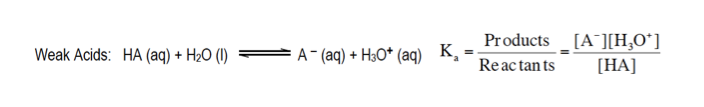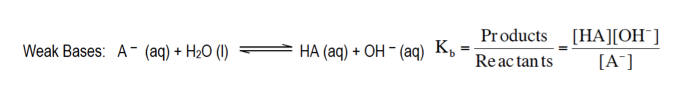Start typing, then use the up and down arrows to select an option from the list.# Analytical Chemistry

Learn the toughest concepts covered in your Analytical Chemistry class with step-by-step video tutorials and practice problems.

Monoprotic Acid-Base Equilibria

# Ka and Kb of compounds

The acid dissociation constant, Ka, and the base dissociation constant, Kb, determine the strengths of weak acids and weak bases respectively.

## Ka and Kb

1

#### example

Ka and Kb4m
Play a video:
mhm. So we're going to say here that associated with any weak acid or any weak base, we have our K and R KB values. So these are equilibrium constants for weak acids and weak bases, respectively. We're gonna say K represents the acid dissociation constant. Um for a weak acid and it measures the strength of weak acids. And we're gonna say here that the KB is representative of our based association constant and it measures the strength of weak bases. We're gonna say in general, the higher your K value is than the lower your P. K. Value is this translates to a stronger acid. So that means that you produce more H plus concentration. So it's gonna increase the concentration of H. Plus, we're gonna say here that generally speaking, weak acids have K values less than one. Weak bases use KB instead they're KB values are less than one now because they're K and KB values are less than one. That means we do not make an appreciable amount of products. So very little product would be made when our weak acid dissolves in solution or when our weak base dissolves in solution. Now, if we're talking about weak acids and weak bases, we're going to say that they establish equilibrium is because they're weak. So for a weak acid here it's the acid water, he will act as the base based on the Bronston Laurie theory, weak acids donate H plus to the base creating a minus and H +30 plus as our products. Here we establish an equilibrium which is the reason for the double arrows. Now, weak acids use K A K A is an equilibrium constant and like all other equilibrium constants, it equals products. Overreact ints remember we ignore solids and liquids. So based on our equilibrium expression would be a minus times H 30 plus over H A. Realize we ignore the water here because it is a liquid. Again, we ignore solids and liquids for weak bases here. It's the base. Water now acts as the acid. It's going to donate an H plus over to the base because according to Bronston Laurie, the acid donates an H plus over to the base, therefore creating a church A and O H minus as my products, weak bases use KB as their equilibrium constant also equals products. Overreact ints again we ignore water because it is a liquid. So these would be our equilibrium expression for a weak acid and for a weak base. Later on we'll learn that through these equilibrium expressions were able to set up an ice chart to determine the ph or P O. H of a weak acid and a weak base respectively. Now K and K B are connected to each other by the formula K W equals K times K B. We've seen K W in the past before. Remember this represents our ion product constant for water and remember at 25 degrees Celsius and equals 1.0 times 10 to the negative 14. This means that at 25 degrees Celsius. If I know K A, I can determine KB because we'll know what KW is and vice versa. If I know K A, I can find KB because again, we know what K W is. So keep in mind this equation here to help you convert from either K two K B or vice versa. Now that we've looked at our descriptions of K and K B. Try to attempt this example question here on the bottom of the page. Um, if you get stuck, don't worry, just click onto the next video and see how I approach that same question which relates acid strength between these two assets. Given an example on the bottom of the page.

Ka examines the strength of weak acids.Kb examines the strength of weak bases.2

#### example

Ka and Kb3m
Play a video:
Okay. So here it says consider to acquit solutions of equal concentration, which statement is true. So we have Cloris acid which has a K. Value of 1.1 times 10 to the negative two. And fennel which has a K. Value of 1.3 times 10 to the negative 10. Alright so both of these assets here would react with water. They both would um donate an H. Plus to water. So we'd have cielo two minus and H +30. Plus being created here. And then here this fennel would also react with water donates an H. Plus to water to produce C. Six H +50. Negative plus H +30. Plus. Now remember the higher your K. Value, the stronger the acid, What does that mean? Well the higher the K. A. The stronger the acid the more it dissociates into these ions products. So basically the higher K. A. The more of these you'll produce, the more products you'll produce. And since Cloris acid has a higher K. Value that means we're going to produce a higher amount of these products. Alright, so now looking at that, let's look and see which statement is true. So porous acid produces more H 30. Plus than fennel. That is true because it has a higher K. Value clark acid is basic compared to phenol. No it's more acidic because it's K. Is larger Cloris acid produces less H 30. Plus than fennel. Know again it produces more H 30. Plus because it's K. Is larger. It's more acidic. Cloris acid is a strong acid. No we said earlier that weak acids have K values less than one. So this would be a weak acid. And then here are chloride ion produces more O. H. Minus than our fan oxide ion. Alright so these are the conjugate base forms of those acids. So let's think about this. So you have cielo too is stronger acid then fennel. If you're stronger as an acid that means you're gonna be weaker as a base. So cielo two minus is a weaker base then C six H 50. Negative. Because remember if you're strong as an asset you're weaker as a base. If you're weaker as a base that means you produce less O. H. Minus. So claudine wouldn't produce more O. H minus would produce less because it is the weaker base of the two. So out of all the choices only option A would be true. So just remember the higher the K. The stronger the acid will be, the more it will produce the desired ions products. But the stronger you are as an acid, the weaker you will be as a base. Remember you can't be incredibly strong both ways. Pick aside your weaker on the opposite. So the stronger the acid, the weaker the base form would be

## Ka and Kb Calculations

3

#### example

Ka and Kb Calculations 11m
Play a video:
Yeah. So here it asks which of the following compounds has the strongest conjugate acid. Now realize that there is give and take if you exist as one of the strongest acids, that means you're gonna exist as also one of the weakest bases. So, strongest conjugate acid here is really saying weakest base. And we're looking at weakest space because all of these compounds here are nitrogenous compounds of nitrogen containing compounds which makes them a means which is the reason they have KB values associated with them. Remember KB values are associated with weak bases. Since we're looking for the strongest conjugate acid, that really means that we're looking at the weakest base, the weakest base would have the smallest KB value. So if we look at all the choices, the one with the smallest KB value would be the last option here. So option d would be our correct choice. So remember if you're incredibly strong one way you're incredibly weak, the opposite. So strongest acid would give us the weakest conjugate base um strong And then we'd say here, strongest base would give me the weakest conjugate acid. So just remember the opposite duality that exists between acids and bases. In order to answer a question like this
4

#### example

Ka and Kb Calculations 12m
Play a video: